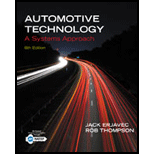Chapter 3, Problem 17RQAutomotive Technology: A Systems A...

6th Edition
Jack Erjavec + 1 other
ISBN: 9781133612315

Solutions

Chapter
SectionAutomotive Technology: A Systems A...

6th Edition
Jack Erjavec + 1 other
ISBN: 9781133612315
Textbook Problem

Torque is a force that does work with a action.

To determine

The appropriate word for the blank in the statement ‘______ action is used by the Torque when work as a force’.

Explanation

Torque may be defined as the effect produced when force acting on a body rotates the body about an axis. In other words, it can produce a twist on the object. It is calculated by multiplying the applied force with the perpendicular distance of the force from the axis of rotation...

Still sussing out bartleby?

Check out a sample textbook solution.

See a sample solution

The Solution to Your Study Problems

Bartleby provides explanations to thousands of textbook problems written by our experts, many with advanced degrees!

Get Started

Find more solutions based on key concepts